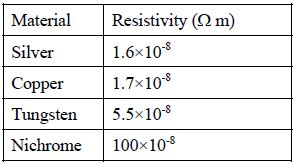# Explain Unit of Specific Resistance

Electrical resistivity (also known as resistivity, specific electrical resistance, or volume resistivity) is an intrinsic property that quantifies how strongly a given material opposes the flow of electric current. Resistivity is the reciprocal of conductivity. A material that has a high resistivity will have a low conductivity. Thus, the specific resistance of essence is the resistance of a unit volume of that matter.

In equation R = ρ (L/A) we can write, ρ = R (A/L)

Substituting the units of the quantities on the right side of the equation, the unit of ρ is:

Ωm2/m = Ωm

Significance: The resistivity of silver at 20°C is 1.6 x 10-8 Ωm. Therefore, the resistance of a silver wire of length lm and a cross-sectional area of 1m2 is 1.6 x 10-8. When referring to an electrical conductor wire of a given cross-section, “specific resistance” can refer to the “resistance per unit length” of the wire, in which case, apparently, its units are “ohm/meter” (Ω/m). Electrical resistance to current flow is the Ohm. SI unit of specific resistance is ohm meter (Ωm)

The table shows the values of the resistivity of some common materials.

Table: Resistivities of different materialsFrom the table above we see that the materials with lower resistivities are good conductors of electricity. For example- copper is the much better conductor of electricity than nichrome. Due to this, copper is widely used as connecting wires in electrical circuits.

In summary, in the corresponding context, it is convenient to use:

• Electrical Resistivity of the material (in Ω m)
• Electrical Resistance per unit length of the wire (in Ω/m)
• Surface Electrical Resistance of the contact (in Ω m2)

Specific resistance is defined as the resistance offered per unit length and unit cross-sectional area when the known amount of voltage is applied. When used to refer to the electrical resistivity of the material, its units are “ohm x meter” (Ωm). In this case, “electrical resistivity” should always be used, instead of the confusing name “specific resistance”. So, it is the resistance in ohms offered by a unit volume (the circular-mil-foot or the centimeter cube) of a substance to the flow of electric current. Resistance to state power is unit less in the SI but the French Resistance did set an enviable standard once.

The resistance of a conductor of a uniform cross-section varies straight as the product of the length and the specific resistance of the conductor, and inversely as the cross-sectional area of the conductor. Therefore, you can evaluate the resistance of a conductor if you know the length, cross-sectional area, and specific resistance of the material.

Besides, materials with higher resistivities also have multiple uses. One example is the nichrome wire. The resistivity and melting point of nichrome is much higher than that of copper. Due to the high resistivity of nichrome, a lot of thermal energy is produced when a current flows through it. This property of nichrome causes water to boil very quickly in the electric kettle. The filament of electric bulbs that are used in our houses is made of tungsten. Tungsten can convert electrical energy to light and thermal energy owing to its high resistivity and melting point.